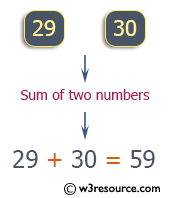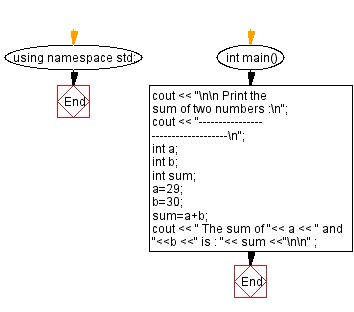﻿ C++ Exercises: Print the sum of two numbers using variables - w3resource

# C++ Exercises: Print the sum of two numbers using variables

## C++ Basic: Exercise-4 with Solution

Write a program in C++ to print the sum of two numbers using variables.

Pictorial Presentation:Sample Solution:

C++ Code :

``````#include <iostream>
using namespace std;

int main()
{
cout << "\n\n Print the sum of two numbers :\n";
cout << "-----------------------------------\n";
int a;
int b;
int sum;
a=29;
b=30;
sum=a+b;
cout << " The sum of "<< a << " and "<<b <<" is : "<< sum <<"\n\n" ;
}
``````

Sample Output:

``` Print the sum of two numbers :
-----------------------------------
The sum of 29 and 30 is : 59
```

Flowchart:C++ Code Editor:

What is the difficulty level of this exercise?

﻿

## C++ Programming: Tips of the Day

Why is there no std::stou?

The most pat answer would be that the C library has no corresponding "strtou", and the C++11 string functions are all just thinly veiled wrappers around the C library functions: The std::sto* functions mirror strto*, and the std::to_string functions use sprintf.

Ref: https://bit.ly/3wtz2qA

We are closing our Disqus commenting system for some maintenanace issues. You may write to us at reach[at]yahoo[dot]com or visit us at Facebook